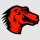Home
IT Knowledge
Inspiration
Languages
EN

# Python - call function of a module by using its name as a string

0 points
Created by:MMORex
300

In this article, we would like to show you how to call a function of a module by using its name as a string in Python.

Quick solution:

``````import math

method = getattr(math, "sqrt")
print(method(16))  # 4.0``````

## Practical example

In this example, we use `getattr()` method to get the `sqrt` method from `math` module.

``````import math

method = getattr(math, "sqrt")
result = method(16)

print(result)   # 4.0``````

Output:

``4.0``